• Call Now

1800-102-2727•

# All About Dimension: Dimensional Formula, Dimensional Analysis, Practice Problems & FAQs

Have you ever thought about how mass and weight are different? Have you ever thought how distance is different from velocity? Every physical quantity has a dimension which represents its constituent fundamental quantities. By dimensional analysis we can understand the significance of a physical quantity and what its unit will be.

Table of content:

• Dimension
• Dimensional formula
• Dimensional Analysis
• List of applications of Dimensional Analysis
• Practice Problems
• FAQs

## Dimension

Dimensions express the nature of a physical quantity. For eg. distance is length. So, its dimension is [L]. The physical quantities can be represented by some combinations of the seven fundamental or base quantities. The seven dimensions of a physical quantity are put inside square brackets. In the dimension of force, it includes 1 dimension in length, -2 dimensions in time and 1 dimension in mass. All other quantities are zero in its dimension. In dimensional analysis, the magnitudes are not considered. Only the type of physical quantity can be recognised through dimension.

In order to express the derived unit of a quantity the fundamental quantities are raised to the powers. The expression thus we get is the Dimension. Let’s understand how!

## Dimensional formula

Dimensional formula is the expression which shows which of the physical quantities are present in the dimension of a physical quantity and how many times a particular dimension is present. The equation obtained by equating the physical quantity with its dimensional formula is called the dimensional equation of the physical quantity

 Quantity Formula Dimension Dimensional formula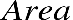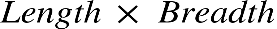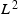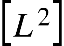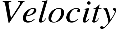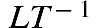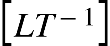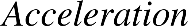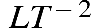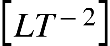In area, the dimension of length is 2.

In velocity, the dimension of length is 1 and the dimension of time is -1.

In acceleration, the dimension of length is 1 and the dimension of time is -2.

The dimension of any physical quantity can be found the same way.

## Dimensional Analysis

It is the practice of checking relations between the physical quantities involved in an equation by identifying the dimensions of the physical quantities.

Dimensions are independent of the numerical multiples and constants. Every quantity in the world can be expressed as functions of the fundamental dimensions.

However, change in units changes the numerical value.

We know that 1000 meters = 1 km,

Therefore,

4 km = 4 × 1000 meters = 4000 meters

Here, the conversion factor is 1000 meters.

## List of applications of Dimensional Analysis

• Checking the correctness of the given physical relation
• Conversion of system of Units
• Deriving the relationship between various physical quantities
• Finding the dimensions of a physical constant or coefficient
• Finding the unit of a physical quantity

## Practice Problems

Q1. Deduce the dimension of Power.

Answer. Power is defined as the rate of work done or rate of energy.

so,

now,

So the dimension would be,

$⇒\left[power\right]=\frac{\left[energy\right]}{\left[T\right]}=\frac{\left[M{L}^{2}{T}^{-2}\right]}{\left[T\right]}=\left[M{L}^{2}{T}^{-3}\right]$

Q2. Deduce the dimension of Angular Momentum.

Answer. Angular momentum is quantified as the product of rotational inertia and angular velocity.

so,

now,

and

So the dimension would be,

Q3. Find whether the dimension of moment and energy are the same or not.

Answer. Energy can be quantified as work done by a force.

So, they both have the same dimension but represent different physical quantities.

Q4. Find the dimension of torque. Torque is the rate of change of angular momentum.

Answer. Torque can be quantified as the rate of change of angular momentum.

so,

## FAQs

Q1. Can a constant have dimension?
Yes, a constant can have dimension. For example

Gravitational constant

$\left[G\right]=\left[{M}^{-1}{L}^{3}{T}^{-2}\right]$

Q2. Which of the following is dimensionless?

a. angle

b. strain

c. specific gravity

d all of the above

Q3. Match the following with same dimensional formula

 a. Impulse 1. Moment b. Heat Energy 2. Momentum c. Specific gravity 3. Work d. Torque 4. Angle

a. a-3, b-2, c-1, d-4

b. a-2, b-1, c-4, d-2

c. a-1, b-3, c-4, d-2

d. a-2, b-3, c-4, d-1

Q4. The dimension of angular momentum is.

a. MLT

b. ML3T-1

c. ML2T-1

d. ML2T-2